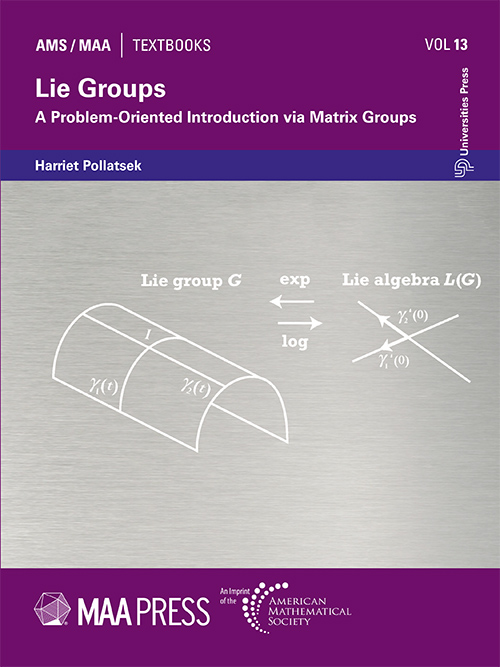## Lie Groups: A Problem-Oriented Introduction via Matrix Groups

#### Price: 895.00

This textbook is a complete introduction to Lie groups for undergraduate students. The only prerequisites are multi-variable calculus and linear algebra. The emphasis is placed on the algebraic ideas, with just enough analysis to define the tangent space and the differential and to make sense of the exponential map. This textbook works, on the principle that students learn best when they are actively engaged. To this end nearly 200 problems are included in the text, ranging from the routine to the challenging level. Every chapter has a section called “Putting the pieces together” in which all definitions and results are collected for reference and further reading is suggested.

Harriet Pollatsek, Mount Holyoke College

Preface
1 Symmetries of vector spaces
1.1 What is a symmetry?
1.2 Distance is fundamental
1.3 Groups of symmetries
1.4 Bilinear forms and symmetries of spacetime
1.5 Putting the pieces together
1.6 A broader view: Lie groups
2 Complex numbers, quaternions and geometry
2.1 Complex numbers
2.2 Quaternions
2.3 The geometry of rotations of R3
2.4 Putting the pieces together
3 Linearization
3.1 Tangent spaces
3.2 Group homomorphisms
3.3 Differentials
3.4 Putting the pieces together
3.5 A broader view: Hilbert’s fifth problem
4 One-parameter subgroups and the exponential map
4.1 One-parameter subgroups
4.2 The exponential map in dimension 1
4.3 Calculating the matrix exponential
4.4 Properties of the matrix exponential
4.5 Using exp to determine L.G/
4.6 Differential equations
4.7 Putting the pieces together
4.8 A broader view: Lie and differential equations
4.9 Appendix on convergence
5 Lie algebras
5.1 Lie algebras
5.2 Adjoint maps|big ‘A’ and small ‘a’
5.3 Putting the pieces together
5.4 A broader view: Lie theory
6 Matrix groups over other fields
6.1 What is a field?
6.2 The unitary group
6.3 Matrix groups over finite fields
6.4 Putting the pieces together
6.5 A broader view: finite groups of Lie type and simple groups
I Linear algebra facts
II Paper assignment used at Mount Holyoke College
III Opportunities for further study
Solutions to selected problems
Bibliography
Index
Notation